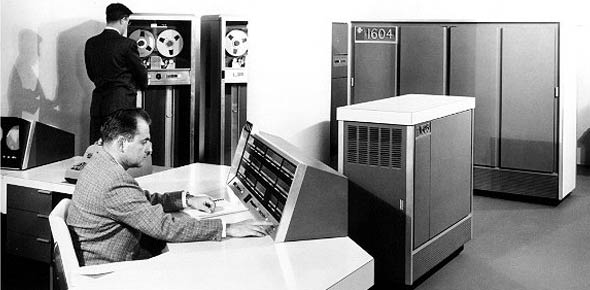# Vol2 Ure1 CDC 3D153

34 Questions | Total Attempts: 71SettingsThis quiz to be used as a study aid for the RF transmission systems 5 level cdc course.

• 1.
(201) Equipment used for generating, amplifying, and transmitting RF carrier is collectively called a?
• A.

• B.

An antenna

• C.

A transmitter

• D.

A transmission line

• 2.
(201) What basic receiver function involves having the transmitted electromagnetic wave pass through the receive antenna in such a manner as to induce a voltage in the antenna?
• A.

Reproduction

• B.

Reception

• C.

Detection

• D.

Selection

• 3.
(201) The ability to reproduce the input signal accurately is the characteristics of the receiver’s?
• A.

Fidelity

• B.

Capacity

• C.

Sensitivity

• D.

Selectivity

• 4.
(202) A combination of a transmitter and receiver that is built as a single unit and sharing common tuned circuits is called a?
• A.

Transmitter

• B.

Transceiver

• C.

• D.

Coupler

• 5.
(202) Which of the following cannot be performed by the transceiver’s computerized components and micro-circuitry?
• A.

Specific equipment faults

• B.

Internal equipment tests

• C.

Internal calibrations

• D.

Repair itself

• 6.
(203) A transmission line that consists of a center conductor placed inside a metal tube functioning as the outer tube is called a?
• A.

Flexible coaxial cable

• B.

Rigid coaxial cable

• C.

Waveguide

• D.

• 7.
(203) Which selection best describes the term “cutoff frequency” when discussing transmission line properties?
• A.

The frequency where XL becomes so low that the signal is shunted.

• B.

The frequency where XC causes the signal to be shunted.

• C.

The lowest frequency that the line can pass successfully

• D.

The frequency at which standing waves are maximum.

• 8.
(203) Using figure 1–10, determine the wavelength if the frequency is changed from 150 MHz to 250 MHz.
• A.

12.0 meters.

• B.

3.0 meters

• C.

1.5 meters

• D.

1.2 meters

• 9.
(203) Using figure 1–10, determine the electrical length if the frequency is changed from 150 MHz to 250 MHz.
• A.

8.33 meters

• B.

8.33 wavelengths

• C.

83.3 meters

• D.

83.3 wavelengths

• 10.
A nonresonant transmission line is a line?
• A.

Having reflected waves

• B.

Having no reflected waves

• C.

With maximum voltage across its open termination

• D.

With maximum voltage across its shorted termination

• 11.
(203) If a transmission line is terminated in a short,
• A.

Current is maximum and voltage at minimum at the termination

• B.

Current is at minimum and voltage at maximum at the termination

• C.

There would be a nominal amount of signal gain

• D.

The line would be nonresonant

• 12.
(204) In a basic communication system, what converts radio frequency (RF) energy’s current oscillation into electric and magnetic fields of force?
• A.

Antenna

• B.

Coupler

• C.

Transmitter

• D.

Transmission lines

• 13.
(204) The concept that alternating current (AC) changes in magnitude and reverses its direction during each cycle is?
• A.

An unproven hypothesis

• B.

The definition of propagation

• C.

• D.

What led to the discovery of direct current

• 14.
(205) If the electric field component travels in a plane perpendicular to the Earth’s surface, the radio wave is?
• A.

Magnetically polarized

• B.

Horizontally polarized

• C.

Circularly polarized

• D.

Vertically polarized

• 15.
(205) What types of polarization do satellite terminals transmit and receive?
• A.

Transmit horizontal and receive vertical polarizations

• B.

Transmit vertical and receive horizontal polarizations

• C.

Transmit right hand and receive left hand circular polarizations.

• D.

Transmit left hand and receive right hand circular polarizations

• 16.
(206) What measurement is used to determine whether an antenna is resonant at a particular frequency?
• A.

Distortion

• B.

Standing Wave Ratio

• C.

Signal-to-noise ratio

• D.

Percent of modulation

• 17.
(206) The ability of an antenna to both receive and transmit equally well is known as the antenna’s?
• A.

Bandwidth

• B.

Resonance

• C.

Reciprocity

• D.

Effectiveness

• 18.
(206) The standard used to measure the radiating effectiveness (gain) of an antenna system is the?
• A.

Marconi antenna

• B.

Isotropic antenna

• C.

Hertz antenna

• D.

Whip antenna

• 19.
(206) What does the effectiveness of an entire transmitting and receiving system depend largely upon?
• A.

Impedance matching

• B.

Antenna distance

• C.

Antenna properties

• D.

Line characteristics

• 20.
• A.

Omnidirectional

• B.

Unidirectional

• C.

Bidirectional

• D.

Circular

• 21.
(207) Which antenna type is usually used on long-range, point-to-point circuits where the concentrated radio energy is needed for circuitry reliability?
• A.

Omnidirectional

• B.

Unidirectional

• C.

Bidirectional

• D.

Circular

• 22.
(208) Ungrounded lengths of wire specifically designed to be either a half-wavelength or more than full wavelength long is called a?
• A.

Reflector

• B.

Hertz antenna

• C.

Marconi antenna

• D.

Vertical monopole

• 23.
(209) A long-wire’s takeoff angle depends on the antenna’s?
• A.

Length

• B.

Directivity

• C.

Front-to-back ratio

• D.

Standing wave ratio

• 24.
(209) All antennas used in satellite communications are designed to be?
• A.

Circular directional

• B.

Omnidirectional

• C.

Bidirectional

• D.

Directional

• 25.
(209) A common satellite antenna is the?
• A.

Whip

• B.

Discone

• C.

Parabolic

• D.

Long-wire

Related TopicsBack to top